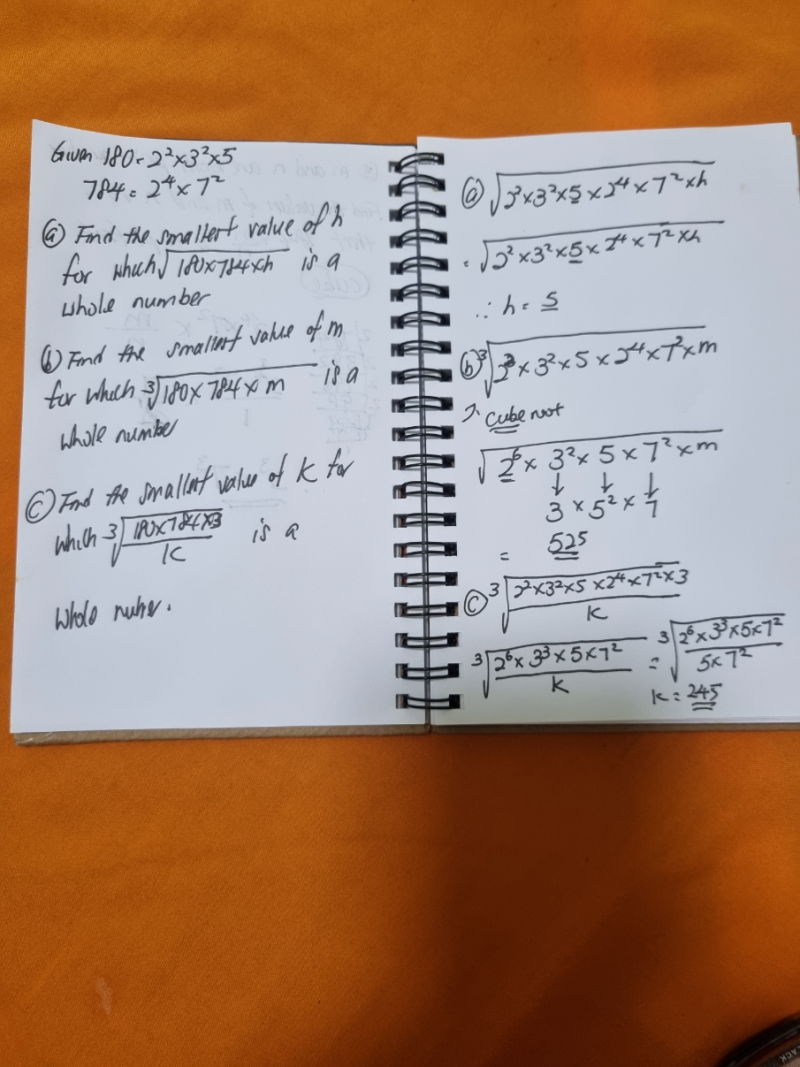# QuestionA more formal way to do the working is this:

```(a)
sqrt(180 x 784 x h) = sqrt(22 x 32 x 5 x 24 x 72 x h)
= 23 x 3 x 7 sqrt(5 x h)
Hence smallest h is 5.

(b)
Let m = 2a x 3b x 5c x 7d.
Then curt(180 x 784 x m) = curt(26+a x 32+b x 51+c x 72+d)
We need exponents (6+a, 2+b, 1+c, 2+d) to be multiples of 3.
The smallest values of (a,b,c,d) is (0,1,2,1) which gives exponents (6,3,3,3).
Hence smallest m = 3 x 52 x 7 = 525.

(c)
Let k = 2a x 3b x 5c x 7d.
Then curt(180 x 784 x 3 / k) = curt(26-a x 33-b x 51-c x 72-d)
We need exponents (6-a, 3-b, 1-c, 2-d) to all be multiples of 3.
The smallest values of (a,b,c,d) is (0,0,1,2) which gives exponents (6,3,0,0).
Hence smallest k = 5 x 72 = 245.```
0 Replies 2 Likes ✔Accepted Answer

A more formal way to do the working is this:

```(a)
sqrt(180 x 784 x h) = sqrt(22 x 32 x 5 x 24 x 72 x h)
= 23 x 3 x 7 sqrt(5 x h)
Hence smallest h is 5.

(b)
Let m = 2a x 3b x 5c x 7d.
Then curt(180 x 784 x m) = curt(26+a x 32+b x 51+c x 72+d)
We need exponents (6+a, 2+b, 1+c, 2+d) to be multiples of 3.
The smallest values of (a,b,c,d) is (0,1,2,1) which gives exponents (6,3,3,3).
Hence smallest m = 3 x 52 x 7 = 525.

(c)
Let k = 2a x 3b x 5c x 7d.
Then curt(180 x 784 x 3 / k) = curt(26-a x 33-b x 51-c x 72-d)
We need exponents (6-a, 3-b, 1-c, 2-d) to all be multiples of 3.
The smallest values of (a,b,c,d) is (0,0,1,2) which gives exponents (6,3,0,0).
Hence smallest k = 5 x 72 = 245.```
0 Replies 2 Likes ✔Accepted Answer